Latest Banking jobs   »   Quantitative Aptitude Quiz For ESIC- UDC,...

# Quantitative Aptitude Quiz For ESIC- UDC, Steno, MTS Prelims 2022-4th January

Q1. A person converted his circular field into a square field by removing some area of circular field. Find the area of square field if area of the circular field is 288 π m² and diameter of circular field is equal to diagonal of square field.
(a) 484 m²
(b) 576 m²
(c) 529 m²
(d) 512 m²
(e) 578 m²

Q2. If 40% of (X + 2000) = 1300 and 60% of (X + Y) = 1830. Find X : Y .
(a) 5 : 8
(b) 20 : 27
(c) 8 : 13
(d) 26 : 35
(e) 25 : 36

Q3. Ratio of P’s age 4 years hence to Q’s age 2 years ago is 4 : 5 and average of their age 3 years ago is 23 years. Age of P five year hence will be?
(a) 25 years
(b) 29 years
(c) 23 years
(d) 33 years
(e) 20 years

Q4. In a mixture of water and alcohol, ratio (water : alcohol) is 8 : 5. When 4 litres of water is added in the mixture, the ratio (alcohol : water) becomes 5 : 9. Find the quantity of initial mixture ?
(a) 39 lit
(b) 104 lit
(c) 52 lit
(d) 78 lit
(e) 91 lit

Q5. The speed of boat in still water is 5 km/hr more than speed of current and the ratio of speed of boat in downstream to the speed of boat in still water is 4 : 3. Find downstream distance covered by boat in 3 hours.
(a) 33 km
(b) 30 km
(c) 36 km
(d) 39 km
(e) 24 km

Q6. A mixture of 30 litres contains alcohol and water in ratio 3 : 7. How much alcohol must be added to this mixture so that the ratio of alcohol and water becomes 2 : 3 ?
(a) 5 ltr.
(b) 6ltr.
(c) 7 ltr.
(d) 4 ltr.
(e) None of these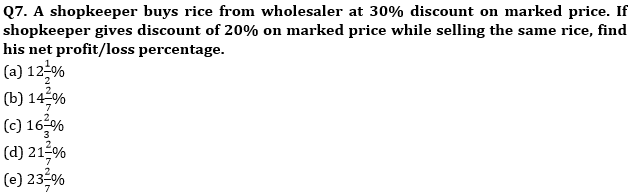Q8. If ratio of length, breadth and height of a cuboid is 1 : 2 : 3 and its area is 88 cm², then find the volume of a cube having edge length equal to the breadth of the cuboid.
(a) 68 cm³
(b) 44 cm³
(c) 64 cm³
(d) 74 cm³
(e) None of these

Q9. The length of train A is twice that of train B and speed of train A is half of that of train B. If train A crosses a man in 4 sec. then find how long will train B take to cross train A if they go in same direction.
(a) 3s
(b) 4s
(c) 5s
(d) 6s
(e) None of these

Q10. The probability of Ram speaking truth is ⅓ and that of Shyam is ⅖. A question is asked from both of them. What is the probability that one of them lies ?
(a) 7/15
(b) 6/15
(c) 5/15
(d) 8/15
(e) None of these

Q11. Veer can do a work in 5 days and Ayush can do the same work in 3 days. If ratio of efficiency of Veer to Ayush to complete the work is (x – 4) : (x + 4), then find the value of x.
(a) 8
(b) 16
(c) 18
(d) 24
(e) 12

Q12. If the difference between CI and SI at the end of 2 years is Rs. 100 then what is the principal given that rate is 5% per annum in both the cases and principal is also the same for both cases.
(a) 35000
(b) 40000
(c) 45000
(d) 36000
(e) 32000

Q13. In an election between two candidates, one got 55% of total valid votes and 20% of the total votes casted were invalid. If total votes were 7500, then what is the number of valid votes that the other person got ?
(a) 2550
(b) 2670
(c) 2700
(d) 2850
(e) 2500

Q14. The price of petrol is increased by 25% by what percent should a car owner reduce the consumption of petrol so that his expenditure on petrol remains constant ?
(a) 18%
(b) 16%
(c) 15%
(d) 20%
(e) 14%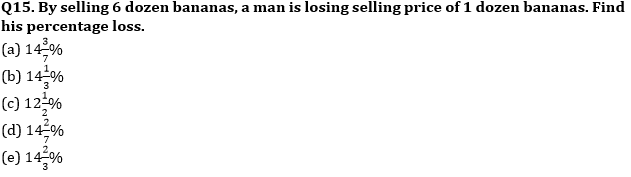Solutions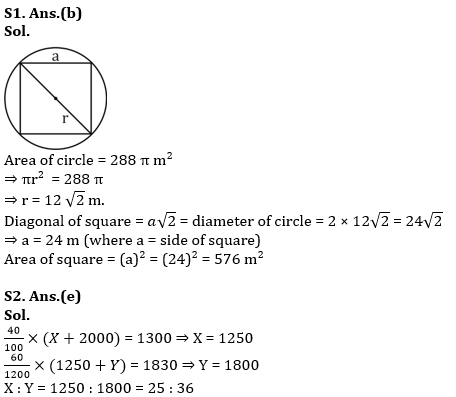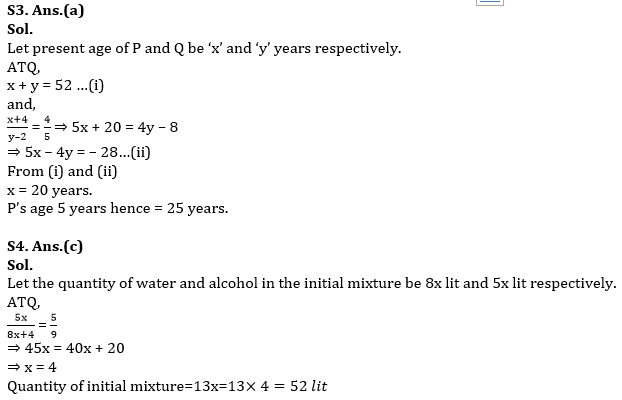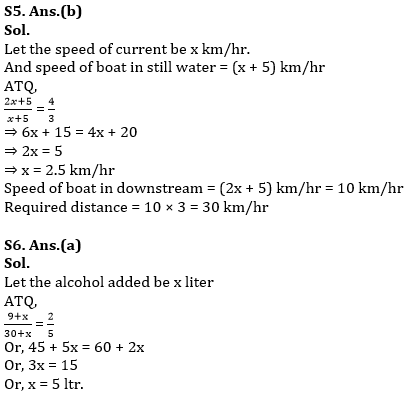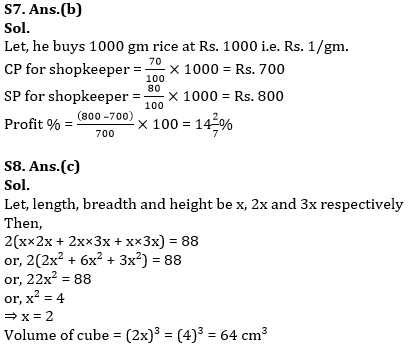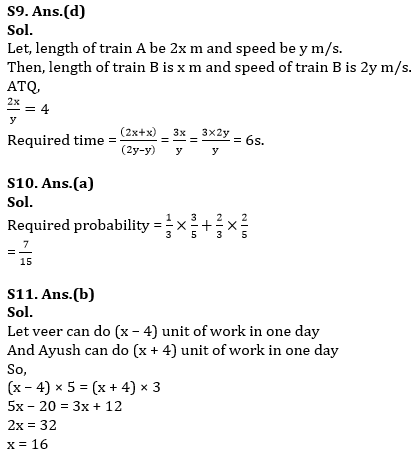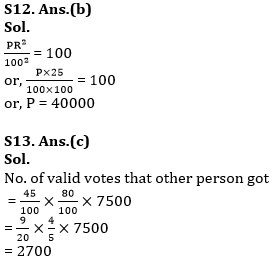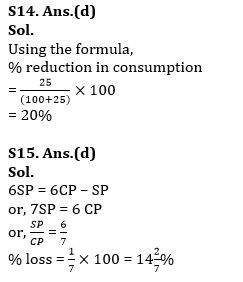#### Congratulations!